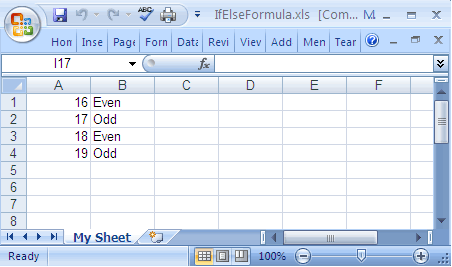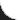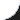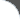Home | Tutorials | Articles | Videos | Products | Tools | Search
 Interviews | Open Source | Tag Cloud | Follow Us | Bookmark | Contact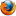In Browser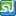StumbleUpon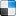del.icio.usGoogle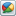Google Buzz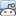redditLinkedInFacebook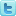TwitterLinkedinE-Mail
 Excel > JExcel API > How to add IfElse Formula to Excel Spreadsheet

Java Excel API is an open source java library to read, write and modify Excel spread sheets. This requires the library jxl-2.6.12.jar to be in classpath. The following example shows how to add ifelse formula to a Cell in Excel Spreadsheet.

 File Name  : com/bethecoder/tutorials/jexcelapi/write/IfElseFormulaTest.java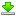Author  : Sudhakar KV Email  : [email protected]

 ``` package com.bethecoder.tutorials.jexcelapi.write; import java.io.File; import java.io.IOException; import jxl.Workbook; import jxl.read.biff.BiffException; import jxl.write.Formula; import jxl.write.Number; import jxl.write.WritableSheet; import jxl.write.WritableWorkbook; import jxl.write.WriteException; import jxl.write.biff.RowsExceededException; public class IfElseFormulaTest {   /**    * @param args    * @throws IOException     * @throws IOException     * @throws WriteException     * @throws BiffException     */   public static void main(String[] args) throws IOException, WriteException {     //Creates a writable workbook with the given file name     WritableWorkbook workbook = Workbook.createWorkbook(new File("C:/JXL/IfElseFormula.xls"));     WritableSheet sheet = workbook.createSheet("My Sheet", 0);     addFormulaCells(sheet, 16, 0);     addFormulaCells(sheet, 17, 1);     addFormulaCells(sheet, 18, 2);     addFormulaCells(sheet, 19, 3);          //Writes out the data held in this workbook in Excel format     workbook.write();      //Close and free allocated memory      workbook.close();    }   private static void addFormulaCells(       WritableSheet sheet,        long num, int row) throws RowsExceededException, WriteException {          //Add number in A - column     Number numCell = new Number(0, row, num);     sheet.addCell(numCell);          String cell = "A" + (row + 1);     String formula = "IF(MOD(" + cell + ",2)=0, \"Even\", \"Odd\")";          //Create a formula for IFELSE     Formula formulaCell = new Formula(1, row, formula);     sheet.addCell(formulaCell);   } }```

It gives the following output,